# Logical Reasoning-Puzzles TNPSC Group 1 Questions

## Logical Reasoning-Puzzles MCQ Questions

13.

From the fig, find the value of 'x'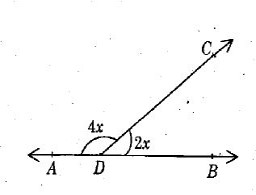Group 4 - 2014

A.

30⁰

B.

60⁰

C.

90⁰

D.

45⁰

A. 30⁰

14.

Find the missing number in the place '?'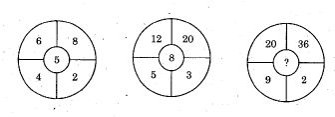Group 4 - 2014

A.

10

B.

11

C.

13

D.

14

D. 14

15.

Find the missing term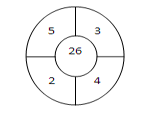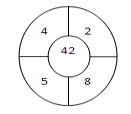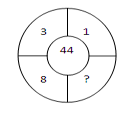Group 2 - 2013

A.

12

B.

18

C.

16

D.

24

A. 12

16.

Find the missing number in the place '?'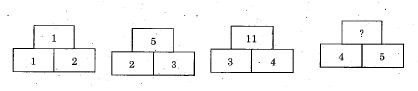Group 4 - 2014

A.

18

B.

19

C.

20

D.

21

B. 19

17.

Find the missing number.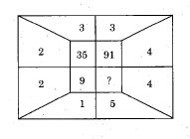Group 4 - 2014

A.

136

B.

156

C.

176

D.

189

D. 189

18.

Which of the following is/are true?

(i) ax = bx => a = b

(ii) x/a =x/b => a = b

(iii) a = b => ax = bx

(iv) a + x = b + x => a = b

VAO - 2014

A.

(i), (ii), (iii) and (iv)

B.

(i), (iii) and (iv) only

C.

(i) and (iv) only

D.

(iii) and (iv) only